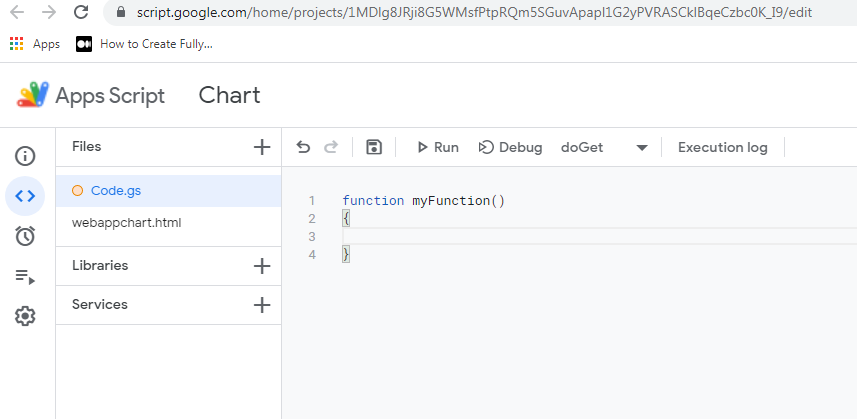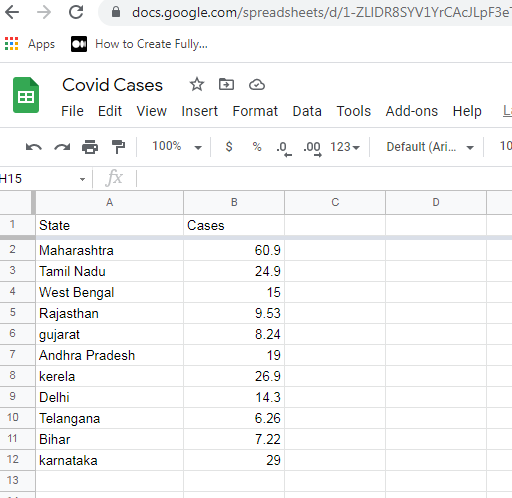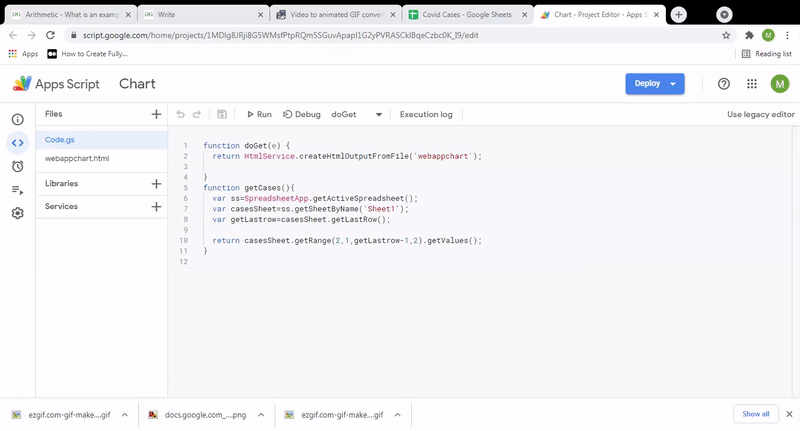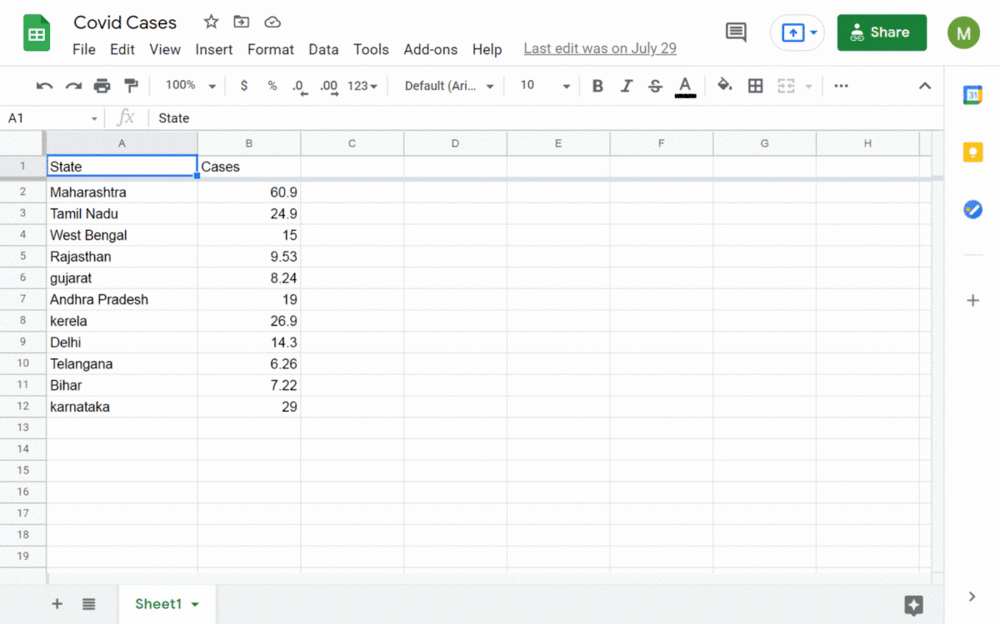Open in App
Not now

• Last Updated : 29 Sep, 2021

In this article, we will be writing a script to create a chart from the data available in Google Sheet. Here, we will be using Chart.js which is a JavaScript library. We are basically developing a web app by publishing the script .

Web App: The script can be published as web app if it contains the function either doGet(e) or doPost(e) and the function must return HTML Service HtmlOutput object. You will learn how to link HTML file to the script in the steps discussed below.

Procedure for writing code in Script Editor:

• Start by creating a new Google Sheet.
• Then click the  Tools tab in the menu as Tools> Script Editor
• A new window will open as shown below:• You can insert your code between the curly braces of the function myFunction block.

Let’s see step by step implementation.

Step 1: Prepare your Google Sheet data. We are having the data of the number of Covid cases in different states in India in lakhs which is as shown below. So, the first step is to prepare your data.Step 2: Add standard Google Apps Script function doGet(e) to code.gs file.  Next step is to go to the tools and select script editor. Save it as ‘Chart’ or any other name.Here we are saving as code.gs . Also create a new HTML file and save it as ‘webappchart.html’ .

Add the below code in code.gs file.

## code.gs

 `function` `doGet(e) {``  ``return` `HtmlService.createHtmlOutputFromFile(``'webappchart'``);``   ` `}`

The above function is the standard Google Apps Script function which is used to publish/deploy your web app. This function returns an output file that is nothing but your HTML file.

Now let’ understand the relation between HTML file and code.gs file:

• HtmlService:
• This class is used as a service to return HTML and other text content from a script
• createHtmlOutputFromFile(filename):
• The above function creates a new HtmlOutput object from a file in the code editor. In our case we have named the file as webappchart.html. So we are using doGet(e) function which returns an HtmlOutput object to our HTML page. Filename should be given in string . The function returns an error if the file is not found.

Step 3: Add the required CDN in your HTML file. Next open your HTML file i.e webappchart.html and we will include the cdn for jQuery and Chartjs which we will be using to make our chart.

<script src=”https://cdnjs.cloudflare.com/ajax/libs/Chart.js/3.4.1/chart.js” integrity=”sha512-lUsN5TEogpe12qeV8NF4cxlJJatTZ12jnx9WXkFXOy7yFbuHwYRTjmctvwbRIuZPhv+lpvy7Cm9o8T9e+9pTrg==” crossorigin=”anonymous” referrerpolicy=”no-referrer”>

Step 4: Create a canvas for the rendering charts. In the next step we complete our html code by adding canvas for displaying our chart inside a div element in the body tag as written below:

## HTML

 `<``div` `style``=``'width:800px height:400px'``>``    ``<``canvas` `id``=``'barchart'` `class``=``'chartjs-render-monitor'``>```

The HTML code is completed over here and now we add the script code.

Step 5: Adding the jQuery Script Code. We initially write the standard jQuery method which is called when the screen is fully loaded with html code and we call getCases() method inside it which we will be defining in the next step.

## Javascript

 `\$(document).ready(``function``(){``      ``getcases();``    ``});`

We then define our getCases() function as below:

## Javascript

 `function` `getCases(){``  ``google.script.run.withSuccessHandler(``function``(ar){``    ``console.log(ar);``    ``var` `data=[];``    ``var` `label=[];``    ``ar.forEach(``function``(item,index){``      ``data.push(item);``      ``label.push(item);``    ``});``  ``});``}`

In the above code we are calling the standard function of Google Apps Script.

Syntax:

``` google.script.run.withSuccessHandler(function(ar)){
}.getCases(); ```

Step 6: Defining function in code.gs file to return data retrieved from Google Sheet. The function getCases which is called over here is defined in the code.gs file as below.Add the below code in code.gs file:

## Javascript

 `function` `getCases(){``  ``var` `ss=SpreadsheetApp.getActiveSpreadsheet();``  ``var` `casesSheet=ss.getSheetByName(``'Sheet1'``);``  ``var` `getLastrow=casesSheet.getLastRow();`` ` `  ``return` `casesSheet.getRange(2,1,getLastrow-1,2).getValues();``}`

Explanation: In the above code we are setting the active spreadsheet to ss and the sheet which is required i.e., Sheet 1 to casesSheet . Then we are getting the last row and setting it in getLastrow variable. Finally we are returning the values from the range given above i.e., starting from second row and so on. An array containing the values from the whole sheet is returned over here and it will be accessible as ar. We are then iterating over the array and pushing the first item in an array as label[] and the second item in an array as data[]. We then will be using these arrays for creating a chart.

Step 7: Creating the chart. Now the data is prepared and we create a bar chart as below:

## Javascript

 `var` `ctx=document.getElementById(``"barchart"``).getContext(``'2d'``);``   ` `var` `barchart=``new` `Chart(ctx, {``  ``type: ``"bar"``,``  ``title:{``    ``display: ``true``,``    ``text: ``"covid cases in different states of India"``  ``},``  ``data : {``    ``labels: label,``    ``datasets: [{``      ``label: ``'Covid cases in different states of India'``,``      ``data: data,``      ``backgroundColor: [``        ``'rgba(255, 99, 132, 0.2)'``,``        ``'rgba(54, 162, 235, 0.2)'``,``        ``'rgba(255, 206, 86, 0.2)'``,``        ``'rgba(75, 192, 192, 0.2)'``,``        ``'rgba(153, 102, 255, 0.2)'``,``        ``'rgba(255, 159, 64, 0.2)'``,``        ``'rgba(255, 99, 132, 0.2)'``,``        ``'rgba(54, 162, 235, 0.2)'``,``        ``'rgba(255, 206, 86, 0.2)'``,``        ``'rgba(75, 192, 192, 0.2)'``,``        ``'rgba(153, 102, 255, 0.2)'``,``        ``'rgba(255, 159, 64, 0.2)'``      ``],``      ``borderColor: [``        ``'rgba(255,99,132,1)'``,``        ``'rgba(54, 162, 235, 1)'``,``        ``'rgba(255, 206, 86, 1)'``,``        ``'rgba(75, 192, 192, 1)'``,``        ``'rgba(153, 102, 255, 1)'``,``        ``'rgba(255, 159, 64, 1)'``,``        ``'rgba(255,99,132,1)'``,``        ``'rgba(54, 162, 235, 1)'``,``        ``'rgba(255, 206, 86, 1)'``,``        ``'rgba(75, 192, 192, 1)'``,``        ``'rgba(153, 102, 255, 1)'``,``        ``'rgba(255, 159, 64, 1)'``      ``],``      ``borderWidth: 1``    ``}]``  ``},``    ` `  ``options: {``    ``legend: {display: ``false``},``    ``title: {``      ``display: ``true``,``      ``text: ``"Covid Cases in India"``    ``}``  ``}``})`

Explanation: In the above code we are assigning number of cases to data and labels are nothing but names of different states in my case. Then we are setting the background color and the border colors of all the bars in our bar chart.

Step 8: Running the WebApp:Now let’s see the complete code of the above implementation.

## code.gs

 `function` `doGet(e) {``  ``return` `HtmlService.createHtmlOutputFromFile(``'webappchart'``);``   ` `}``function` `getCases(){``  ``var` `ss=SpreadsheetApp.getActiveSpreadsheet();``  ``var` `casesSheet=ss.getSheetByName(``'Sheet1'``);``  ``var` `getLastrow=casesSheet.getLastRow();`` ` `  ``return` `casesSheet.getRange(2,1,getLastrow-1,2).getValues();``}`

## index.html

 ```<``html``>`` ` `<``head``>``  ``<``base` `target``=``"_top"``>``  ``<``script` `src``=``"https://ajax.googleapis.com/ajax/libs/jquery/3.6.0/jquery.min.js"``>``  ````  ``<``script` `src``=``"https://cdnjs.cloudflare.com/ajax/libs/Chart.js/3.4.1/chart.js"``    ``integrity``=``"sha512-lUsN5TEogpe12qeV8NF4cxlJJatTZ12jnx9WXkFXOy7yFbuHwYRTjmctvwbRIuZPhv+lpvy7Cm9o8T9e+9pTrg=="` `    ``crossorigin``=``" anonymous"` `    ``referrerpolicy``=``"no-referrer"``>``  `````` ` `<``body``>``  ``<``div` `style``=``'width:800px; height:400px;'``>``    ``<``canvas` `id``=``'barchart'` `      ``class``=``'chartjs-render-monitor'``>``    ````  ```` ` `  ``<``script``>``    ``\$(document).ready(function () {``      ``getcases();``    ``});``     ` `    ``function getcases() {``      ``google.script.run``        ``.withSuccessHandler(function (ar) {``          ``console.log(ar);``          ``var data = [];``          ``var label = [];``           ` `          ``ar.forEach(function (item, index) {``            ``data.push(item);``            ``label.push(item);``          ``});`` ` `          ``var ctx = document.getElementById(``            ``"barchart").getContext("2d");``                 ` `          ``var barchart = new Chart(ctx, {``            ``type: "bar",``            ``title: {``              ``display: true,``              ``text: "covid cases in different states of India",``            ``},``            ``data: {``              ``labels: label,``              ``datasets: [{``                ``label: "Covid cases in different states of India",``                ``data: data,``                ``backgroundColor: [``                  ``"rgba(255, 99, 132, 0.2)",``                  ``"rgba(54, 162, 235, 0.2)",``                  ``"rgba(255, 206, 86, 0.2)",``                  ``"rgba(75, 192, 192, 0.2)",``                  ``"rgba(153, 102, 255, 0.2)",``                  ``"rgba(255, 159, 64, 0.2)",``                  ``"rgba(255, 99, 132, 0.2)",``                  ``"rgba(54, 162, 235, 0.2)",``                  ``"rgba(255, 206, 86, 0.2)",``                  ``"rgba(75, 192, 192, 0.2)",``                  ``"rgba(153, 102, 255, 0.2)",``                  ``"rgba(255, 159, 64, 0.2)",``                ``],``                ``borderColor: [``                  ``"rgba(255,99,132,1)",``                  ``"rgba(54, 162, 235, 1)",``                  ``"rgba(255, 206, 86, 1)",``                  ``"rgba(75, 192, 192, 1)",``                  ``"rgba(153, 102, 255, 1)",``                  ``"rgba(255, 159, 64, 1)",``                  ``"rgba(255,99,132,1)",``                  ``"rgba(54, 162, 235, 1)",``                  ``"rgba(255, 206, 86, 1)",``                  ``"rgba(75, 192, 192, 1)",``                  ``"rgba(153, 102, 255, 1)",``                  ``"rgba(255, 159, 64, 1)",``                ``],``                ``borderWidth: 1,``              ``},``            ``],``            ``},`` ` `            ``options: {``              ``legend: { display: false },``              ``title: {``                ``display: true,``                ``text: "Covid Cases in India",``              ``},``            ``},``          ``});``          ``})``          ``.getCases();``        ``}``    `````` ` ``

Output:My Personal Notes arrow_drop_up